Courses

# Short & Long Answer Question(Part-1) - Chemical Bonding and Molecular Class 11 Notes | EduRev

## JEE : Short & Long Answer Question(Part-1) - Chemical Bonding and Molecular Class 11 Notes | EduRev

The document Short & Long Answer Question(Part-1) - Chemical Bonding and Molecular Class 11 Notes | EduRev is a part of the JEE Course Chemistry for JEE.
All you need of JEE at this link: JEE

Q.1 A molecule of H2 exists while that of the He2  does not. Explain.

Answer: Hydrogen atom (Z = 1) has only one electron present in the s-orbital(1s1). The two such half filled atomic orbitals combine to form a molecular orbital which contains both these electrons. But helium (Z = 2) has already a filled orbital (1s2). Therefore, the atomic orbitals of the helium atoms do not combine. Thus, a molecule of  H2  exists while that of  He2 does not.

Q.2 NaCl gives a white precipitate with AgNO3  solution but CCldoes not. Why?

Answer: Both NaCl and AgNO3 are ionic solids and they readily dissociate to form ions in solution. Ag+ions combine with Cl ions to give white precipitate of AgCl. But CCl4 is covalent compound and does not give Cl  ions in solution. Therefore, no reaction is possible between CCl4 and AgNO3.

Q.3 BeFand H2O are both tri-atomic molecules but have different shapes. Discuss.

Answer: According to VSEPR theory, the geometry and shape of a covalent molecule does not depend upon the number of atoms but on the number of the electron pairs around the central atom. Molecules with different number of such electron pairs are expected to have different shapes. In BeF2, the Be atom is surrounded by two electron pairs while four pairs are present around O atom in H2O. Therefore, they have different shapes.

Q.4 Bond angle in NH3 is more than in PH3. Explain.

Answer: Both NHand PH3 are the hydrides of nitrogen and phosphorus which are present in group 15. Nitrogen is more electronegative (3.0) as compared phosphorus (2.1). As a result, the shared electron pairs lie closer to the nitrogen atom in NH3 than to the phosphorus atom in PH3. Therefore, the electron density around the nitrogen atom is more than around the phosphorus atom. This results in greater repulsion in the electron pairs around nitrogen atom than around phosphorus atom. As a result, bond angle in NH3  is more than in PH3.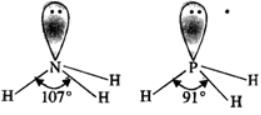Q.5 Why is HCl predominantly covalent in the gaseous state but is ionic in aqueous solution?

Answer: In HCl, the electro negativity difference in Cl and H atoms (3.0 - 2.1) is 0.9. Therefore, it is predominantly covalent in the gaseous state. When dissolved in water which is of polar nature, there is an attraction between the atoms of the acid and polar H2O molecules. Energy is released during attraction. This helps in the ionisation of the acid. Therefore, HCl is ionic in aqueous solution.

Q.6 Can a non-polar molecule have polar covalent bonds?

Answer: Yes, it is possible in case of molecules having linear and symmetrical shapes. In them, the bonds are polar but their polarities cancel out resulting in non-polar molecules. For example, in CO2(O=C=O) both the bonds are polar. But as it is linear in nature, the polarities of the bonds cancel and the molecule is non-polar in nature.

Q.7 Sigma bond is stronger than the pi bond. Explain.

Answer: The strength of a covalent bond depends upon the extent of the overlap. Greater the overlap, stronger is the bond. A sigma bond is formed by the axial overlap of the orbitals while pi bond by their sidewise or lateral overlap. Since the axial overlap is more effective than the sidewise overlap, greater energy is will be released in the axial overlap as compared to sidewise overlap. Therefore, sigma bond a stronger than the pi bond.

Q.8 A molecule of PCl5 exists while that of NCl5  does not.
The valence shell electronic configurations of both N and P are as follows :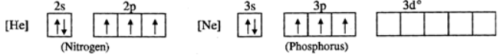Since nitrogen atom has no vacant 2d orbitals, it cannot extend its covalency to five. Therefore, a molecule of NCl5 does not exist. But in case of phosphorus, an electron can be promoted from 3s filled orbital to 3d vacant orbitals. Therefore, phosphorus can extend its covalency to five. Thus, a molecule of PCl5 can exist.

Q.9  o-nitrophenol is steam volatile while p-nitrophenol is not. Discuss.
In o-nitrophenol, intra molecular hydrogen bonding is present while inter molecular hydrogen is present in the molecules of p-nitrophenol. Energy is needed to overcome attractive forces in the molecules of p-nitrophenol but no such energy is required in case of molecules in o-nitrophenol. This means that the boiling point of o-nitrophenol is less and is steam volatile while that of p-nitrophenol is more and is, therefore, non-volatile in steam.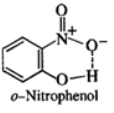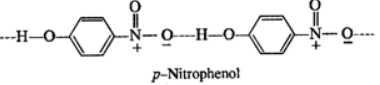Q.10 KHF2 exists while KHCl2 does not. Explain.

Answer: HF molecules are hydrogen bonded as H−F....H−F...H−F....  Therefore, they can associate to form HF2 anion which can exist in combination with K+ ion as KHF2. But no hydrogen bonding is present in H−Cl molecules. Therefore the anion HCl2 and KHCl2  do not exist.

Q.11 You are given the electronic configuration of five neutral atoms - A, B, C, D and E. A−1s22s22p63s2  ;  B−1s22s22p23s1;    C−1s22s22p1;    D−1s22s22p5;    E−1s22s22p6. Write the empirical formula for the substances containing (i) A and D (ii) B and D (iii) only D (iv) only E.

Answer: (i) The empirical formula for the compound containing A and D is AD2. This is because atom A has two valence electrons while D has seven electrons. Therefore, A donates two electrons to two atoms of D and the compound has the formula AD2. (ii) The empirical formula for the compound is BD because B loses one electron while D accept it. (iii) Two atoms of D can share one electron each to complete their octet. Therefore, the empirical formula of the compound is D2. (iv) Since E has the configuration of a noble gas (Ne), it is, therefore, mono atomic and exists as E.

Q.12 Boiling point of ethane is more than that of methane. Assign reason.

Answer: The molecular size of ethane (CH3−CH3)  molecule is more than that of methane(CH4). Therefore, the van der Waals forces of attraction are more in ethane molecules than in molecules of methane resulting in higher boiling point.

Q.13 Which compound from each of the following pairs is more covalent and why? (a) CuO or CuS (b) AgCl or AgI (c) PbCl2 or PbCl(d) BeCl2 or MgCl2

Answer: This is explained with the help of Fazan's rules (a) CuS is more covalent than CuO because S2− ion is more polarised than the O2− ion due to bigger size. (b) AgI is more covalent than AgCl  because I ion is more polarised than Cl ion due to bigger size. (c)PbClis more covalent than PbClbecause Pb4+ ion due to smaller size and greater magnitude of positive charge is more polarising than Pb2+ion. (d)BeCl2 is more covalent than MgCl2 because the Be2+ ion is more polarising than Mg2+ ion due to smaller size.

Q.14 The bond angles in NH+4  and CH4 are same but NH3 has different bond angle. Why?

Answer: In NH+4 ion as well as in CH4, the central atom has four shared pair of electrons. Therefore, both of them have almost the same bond angle (109°28'). But in NH3  molecule, due to the presence of three shared pairs and one lone pair, the geometry gets slightly distorted. As a result, the bond angle is 107.

Q.15 Predict which out of the following species are planar. (i)NH+4 (ii)CH+3 (iii)SF4 (iv)OF (v)H2O

Answer: The species will be planar if the central atom is sp2 hybridised. Let us calculate the hybridisation in all these species.
(i)NH+4:H=1/2[5+4−1+0]=4 (sp3-non-planar)
(ii)CH+3:H=1/2[4+3−1+0]=6/2=3 (sp2- planar)
(iii)SF4:H=1/2[6+4−0+0]=10/2=5 (sp3d- non-planar)
(iv)OF2:H=1/2[6+2−0+0]=8/2=4 (sp3- non-planar)
(v)H2O:H=1/2[6+2−0+0]=8/2=4 (sp3-non-planar)

Q.16 Why is MgCl2 molecule linear whereas the molecule of SnCl2 chloride has angular shape?

Answer: Let us find the hybridisation of central atom in both these molecules. In MgCl2, hybridisation of Mg=1/2[2+2−0+0]=4/2=2(sp) In SnCl2, hybridisation of Sn=1/2[4+2−0+0]=6/2=3(sp2) Thus, MgCl2 is linear while SnCl2  is expected to have planar geometry. However, the presence of a lone pair of electrons on Sn  atom makes it angular.

Q.17 The hybridisation of oxygen in both water and diethyl ether molecules is the same but they differ in their bond angles. Explain.

Answer: In both the molecules, the oxygen atom is sp3 hybridised and the expected bond angle is 109∘28′.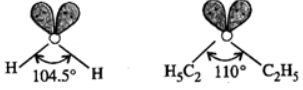However, the presence of two lone pairs distorts the geometry of both these molecules. The bond angle in diethyl ether molecule is more than in case of water molecule because of greater repulsion in two C2H5 groups as compared to H atoms in the molecule of water.

Q.18 Both Na and H occur in group 1 of the periodic table ; yet melting point of NaCl is 800C while that of HCl  is−114C. Why?

Answer: Though Na and H are present in the group 1, they differ in their electro negativities. The electro negativity of H is 2⋅1 and that of Na is 0⋅9. Due to large electro negativity difference between Na and Cl(3⋅0−0⋅9=2⋅1),NaCl  is ionic in nature and exists as a crystalline solid with very high melting point (800°C). On the other hand, HCl is polar covalent due to small electro negativity difference(3⋅0−2⋅1=0⋅9) and exists in the gaseous state at room temperature. In order to solidify it, a very low temperature (−114C) is needed.

Q.19 Write the Lewis dot symbols and predict the valencies you expect for the following elements: Nitrogen, Fluorine and Neon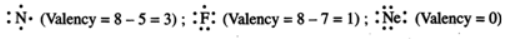Q.20 Out of NaCl and MgO, which has higher lattice energy and why?

Answer: MgO has higher lattice energy because each ion carries two unit charge whereas in NaCl each ion carries one unit charge

Q.21 Out of MgO, and CaO, which one is more hard and why?

Answer: MgO, is harder than CaO. This is because Mg2+ ions are smaller in size than Ca2+ ions. Hence, lattice energy of MgO is greater.

Q.22 Why an ionic bond is formed between two elements having large difference in their electro negativity?

Answer: The more electronegative element will attract the shared pair of electrons to such a large extent than the other that it will amount to transfer of electron resulting in the formation of ions.

Q.23 Is CaF2 linear or bent or neither of the two? Justify.

Answer: CaF2 is neither linear nor bent molecule because it is ionic compound and ionic bond is non-directional.

Q.24 Why NaCl gives a white precipitate with AgNO3 solution but CCl4 does not?

Answer: NaCl is an ionic compound and hence gives Cl ions in the solution which combine with Ag+ ions given by AgNOto form a white precipitate of AgCl but CCl4 is a covalent compound and does not give Clions.

Q.25 Define lattice enthalpy. How is it related to the stability of an ionic compound?

Answer: Definition-see page 4/7. Greater the lattice enthalpy of an ionic compound, greater is the stability.

Q.26 Why is solubility of MgCl2 much greater than that of MgF2?

Answer: Cl ions are much larger in size that F ions. Therefore, lattice enthalpy of MgCl2 is much smaller than that of MgF2 and the solubility is more.

Q.27 Draw the Lewis structures of the following molecules and ions and tell in which case/cases the octet rule is violated CO2,SO2,BeCl2,NH3,AlCl3,PCl5,CO2−3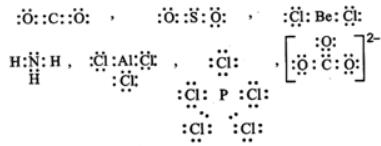The octet rule is violated in case of BeCl2,AlCl3 and PCl5.

Q.28  On the basis of VSEPR theory, predict the shapes of the following molecules/ions? (i) SiF4 (ii) NH(iii) NH+4 (iv) C2H2 (v) H3O+(vi) F2O (vii) PCl3 (viii) PF5.

Answer: (i) Tetrahedral (ii) V-shape (lii) Tetrahedral (iv) Cylindrical (v) Trigonal pyramidal (vi) V-shape (vii) Trigonal pyramidal (viii) Trigonal bipyramidal.

Q.29 Arrange the following in order of decreasing bond angle, giving reason : NO2,NO+2,NO2

Answer: NO+2>NO2>NO2. This is because NO+2 has no lone pair of electrons (i.e. has only bond pairs on two sides) and hence it is linear. NO2 has one unshared electron while NO2 has one unshared electron pair. There are greater repulsions on N−O bonds in case of NOthan in case of NO2.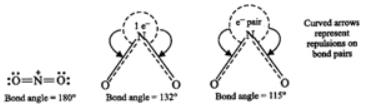Q.30  Explain how the valence bond theory accounts for (i) a carbon-carbon double bond (C = C)    (ii) a carbon-carbon triple bond (C≡C)

• double bonda covalent bond in which two electron pairs (instead of the usual one) are shared between two atoms; most common between carbon atoms and carbon, oxygen, or nitrogen atoms
• pi bondcovalent chemical interactions where two lobes of one involved atomic (p) orbital overlap two lobes of the other involved atomic (p) orbital
• triple bonda covalent bond in which three electron pairs (instead of the usual one) are shared between two atoms; most common between carbon atoms and carbon or nitrogen atoms; symbolized in formulae as ≡

Q.31 Explain how VB theory differs from Lewis concept.

Answer: (i) According to Lewis concept, a covalent bond is formed by mutual sharing of electrons whereas according to VB theory, a covalent bond is formed by the overlap of half-filled atomic orbitals containing electrons with opposite spin. (ii) VB theory can explain the shapes of molecules whereas Lewis concept cannot. (iii) VB theory can explain the strength of bonds whereas Lewis concept cannot.

Q.32 Out of σ and π-bonds, which one is stronger and why?

Answer: σ-bond is stronger. This is because σ-bond is formed by head-on overlapping of atomic orbitals and, therefore, the overlapping is large. π-bond is formed by sideway overlapping which is small.

Q.33 What order of C−H bond lengths do you expect in C2H6,C2H4 and C2H2 and why?

Answer: C−H(C2H6)>C−H(C2H4)>C−H(C2H2). This is because hybrid orbitals of carbon involved in overlapping with 1 s orbital of hydrogen are sp3,sp2  and sp respectively and their sizes are in the order sp3>sp2>sp .

Q.34 Which bond do you expect to be stronger in each of the following cases and why? (i) H−H,Cl−Cl (ii) O2,N2 (iii) F−F,Cl−Cl

Answer: (i) H-H is stronger because of smaller size of H-atoms. (ii)N≡N is stronger because it contains a triple bond while O2 contains a double bond (O=O). (iii) Cl−Cl is stronger because repulsions between the two F-atoms in F2 are larger on account of greater electron density around F-atom due to smaller size and hence greater repulsions between the two F-atoms.

Q.35 Arrange the following single bonds in order of bond energy giving reasons: C - C, N - N, O - O, F - F

Answer: C-C > N-N > O-O > F-F .

Q.36 Explain why dipole moment of hydrogen halides decreases from HF to HI.

Answer: This is because electro negativity of halogen atom decreases from F to I. Hence, the polar character decreases and so is the dipole moment.

Q.37 Represent diagrammatically the bond moments and the resultant dipole moments in (i) SO2 (ii) cis and trans forms of C2H2Cl2

(i)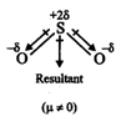(ii)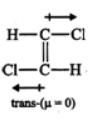(iii)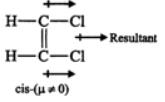Q.38 Predict which out of the following molecules will have higher dipole moment and why? CS2 and OCS

Answer: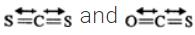both are linear molecules but bond moments in CS2 cancel out so that net dipole moment = 0. But in OCS, bond moment of C = O is not equal to that of C = S. Hence, it has a net dipole moment. Thus, dipole moment of OCS is higher.

Q.39  Indicate whether the following statement is TRUE or FALSE.  Justify your answer in not more than three lines: The dipole moment of CH3F is greater than that of CH3Cl

Answer: False because no doubt C−F bond is more polar than C−Cl bond due to greater electro negativity of F than Cl but C ? F bond length is much smaller than C−Cl bond length.

Q.40 Which is more polar CO2 or N2O? Give reason.

Answer: N2O is more polar than CO2. This is because CO2 is linear and symmetrical. Its net dipole moment is zero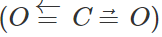.N2O  is linear but unsymmetrical. It is considered as a resonance hybrid of the following two structures: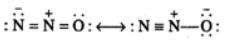It has a net dipole moment of 0.116 D.

Q.41 Why reaction between NaCl and AgNO3 is very fast but reaction between H2 and Clis slow?

Answer: NaCl and AgNOare ionic compounds. In solution, NaCl gives Na+ and Cl ions and AgNO3 gives Ag+and NO3 ions. The oppositely charged Ag+ and Cl ions combine immediately. The reaction between H2and Cl2 involves breaking of covalent bonds which requires energy.

Q.42  Why AlF3 is a high melting solid whereas SiF4 is a gas?

Answer: AlFis an ionic compound whereas SiF4 is a non-polar covalent compound. Hence, inter particle forces in AlF3 are much stronger.

Q.43 Draw the shapes of the following hybrid orbitals ; sp,sp2 and sp3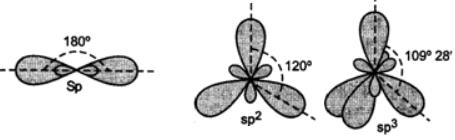All the hybrid orbitals have same shape. However, their sizes are in the order sp<sp2<sp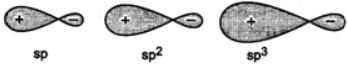Q.44  Name the type of hybridisation of each C-atom in a molecule of (i) propylene (propene) (ii) propyne. How many σ and π-bonds are present in each case?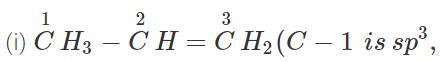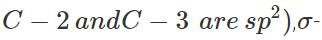bonds =8,π-bonds = 1. (ii)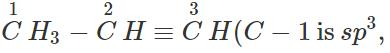C−2and C−3 are sp), σ- bonds = 6, π-bonds = 2.

Q.45 Why NF3 is pyramidal but BF3 is triangular planar?

Answer: In NF3,N has the hybridisation sp3 with one position occupied by lone pair of electrons but in BF3,B has the hybridisation sp2.

Q.46 Out of p-orbital and sp-hybrid orbital which has greater directional character and why?

Answer: sp-orbital has greater directional character than p-orbital. This is because p-orbital has equal sized lobes with equal electron density in both the lobes whereas sp-hybrid orbital has greater electron density on one side.

Q.47 What angles are associated with the following orbitals? sp,sp2 and sp3
: sp=180∘,sp2=120,sp3=10928′.

Q.48 Which d-orbital is involved in sp3d hybridisation and why?

Answer: dz2. The three planar triangular hybrid orbitals may be assumed to be formed from one s and two p-orbitals (sp2) and the remaining p-orbital may combine with dz2 to form two axial hybrid orbitals.

Q.50 Which d-orbital is involved in dsp2 hybridisation and why?

Answer: dx2−y2. This is because its four lobes lie along the x-axis and y-axis. The two p-orbitals can combine along these axes.

Offer running on EduRev: Apply code STAYHOME200 to get INR 200 off on our premium plan EduRev Infinity!

## Chemistry for JEE

223 videos|452 docs|334 tests

,

,

,

,

,

,

,

,

,

,

,

,

,

,

,

,

,

,

,

,

,

;